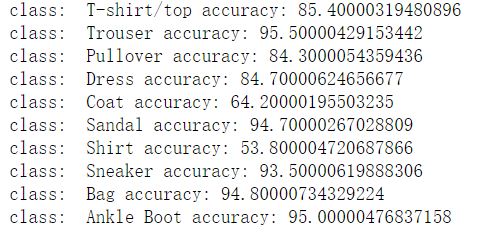# What does ground truth stand for

I am facing one confusing problem when I worked on “VISUALIZING MODELS, DATA, AND TRAINING WITH TENSORBOARD” of pytorch tutorial.
At the sixth part called “Assessing trained models with TensorBoard”, I found something confusing about SummaryWriter.add_pr_curve() function.(the code of the tutorial is showed in the picture )

The second parameter of this function in the tutorial is whether the predictions of testset through the trained network equals class for which we are tring to plot PR curve, however
,according to the documentaions, the second parameter stands for the ground truth. From my perspective, the second parameter of the function in the tutorial should be “testset.targets==class_index”, since ground truth should be whether the labels of the test set , rather than the predictions of testset, equal the class for which we are tring to plot PR curve.
However, after I try this in my code, I got terrible result of PR curve for each class(result is showed in the picture) and when I just run the code in the tutorial, I got wonderful PR curve.
Could someone help me and explain what the ground truth means in this function? Do I have wrong understand of the ground truth?
I would appreciate every response toward this question

this is esults when I turned the code to“testset.targets==class_index”

And I also figure out every class’s accuracy to show that the network is trained appropriately.`class_index` is assigned to the current class index in the for loop over all classes.
Your code (`testset.targets == class_index`) wouldn’t use the model predictions, but just all targets and the current selected class target.

The function will plot a PR curve for each class.

EDIT: Issue might be related to this post.

I really appreciate your reply